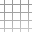#Jan Varho

## Ray-Sphere Intersection

I'm writing a small raytracer to familiarize myself with Java. Obviously many of the main algorithms need to be as fast as possible in order to eventually get to real time speeds.

Here's my optimized version of a ray-sphere intersect check. It can probably be tweaked further: 9 additions, 7 multiplications best case; and 12 additions, 7 multiplications and a square root worst case is the current speed/complexity.

The method only calculates t - the coefficient of the ray direction vector. That's because there is no use calculating the actual intersect position before the smallest t has been found.

Feel free to use however you like.

` public double intersectRay(double[] ro, double[] rd, double minDist) { double px = ro-position; double py = ro-position; double pz = ro-position; double b0 = rd*px + rd*py + rd*pz; double d0 = b0*b0 + size - px*px - py*py - pz*pz;`

// No intersect if (d0<0) return 0;

b0 = -b0; d0 = Math.sqrt(d0); double t = b0 - d0; if (t > EPSILON) { // t0

// Too far if (t > minDist) return 0; } else { // t1 t = b0 + d0;

// Behind or too far if (t <= EPSILON || t > minDist) return 0; }

// Valid intersect return t; }</code>